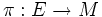# Sheaf of sections of a vector bundle

Let$M$ be a differential manifold, and$\pi:E \to M$ be a smooth vector bundle over$M$. The sheaf of sections of$E$ is defined as follows:
• To every open subset$U$ of$M$, it associates the vector space of all smooth sections of the bundle$\pi^{-1}(U) \to U$